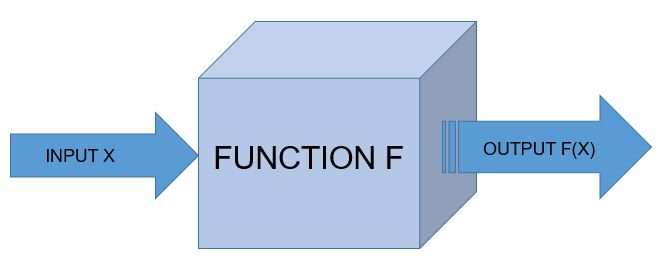# Functions and Built-in Functions in PythonFunction

A function is the simplest callable object in Python.

NOTE: For Indentation either use TAB space or 4 space characters, never both.

def tax(bill):
“””Adds 25% tax to a restaurant bill.”””
bill *= 1.25
print “With tax: %f” % bill
return bill

def tip(bill):
“””Adds 35% tip to a restaurant bill.”””
bill *= 1.35
print “With tip: %f” % bill
return bill

meal_cost = 100
meal_with_tax = tax(meal_cost)
meal_with_tip = tip(meal_with_tax)

Function Syntax

“”” This is spam function”””            /* Comment*/
print “Spaceship!”                            /*body*/

spam()

Call and Response

def square(n):
“””Returns the square of a number.”””
squared = n**2
print “%d squared is %d.” % (n, squared)
return squared

square(5)

Parameters and Arguments

A function can require as many parameters as you’d like, but when called the function, it should generally pass in a matching number of arguments.

result = base**exponent
print “%d to the power of %d is %d.” % (base, exponent, result)

Functions Calling Functions

A function can call another function.

def n_plus_one(n):
return n + 1

return n_plus_one(n) + 2

Importing Module

A module is a file that contains definitions, including variables and functions, that we can use once it is imported.

import math
print math.sqrt(25)

math includes a number of useful variables and functions, and sqrt() is one of those functions. In order to access math, all you need is the import keyword. When you simply import a module this way, it’s called a generic import.

It’s possible to import only specific variables or functions from a given module. Pulling in just a single function from a module is called a function import.

# Import *just* the sqrt function from math

from math import sqrt

if we want all the variables and functions in a module but don’t want to have to constantly type math then we can use

# Import *everything* from the math module

from math import *

Built-in Functions

max()

The max() function takes any number of arguments and returns the largest one.

>> maximum = max(10,35,64)
>> print maximum

min()

min() function returns the smallest of a given series of arguments.

>> minimum = (10,35,64)
>> print minimum

abs()

The abs() function returns the absolute value of the number it takes as an argument, it gives the number’s distance from 0 on an imagined number line.
>> absolute = abs(-42)
>> print absolute

type()

The type() function returns the type of the data it receives as an argument.

>> print type(42)
>> print type(4.2)
>> print type(‘spam’)

Now let us see the code which returns biggest, smallest and absolute values.

def biggest_number(*args):
print max(args)
return max(args)

def smallest_number(*args):
print min(args)
return min(args)

def distance_from_zero(arg):
print abs(arg)
return abs(arg)

biggest_number(-105, -53, 51, 100)
smallest_number(-106, -55, 54, 105)
distance_from_zero(-104)

These are some of the functions and built-in functions in python.